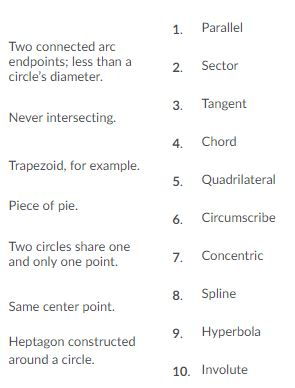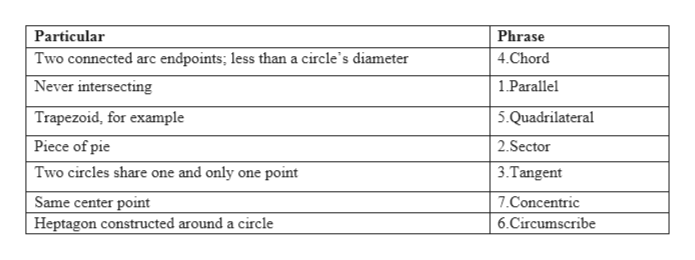# 1. Parallel Two connected arc endpoints; less than circle's diameter. 2. Sector 3. Tangent Never intersecting. Chord Trapezoid, for example. 5. Quadrilateral Piece of pie. 6 Circumscribe Two circles share one 7. Concentric and only one point. 8. Spline Same center point. 9 Hyperbola Heptagon constructed around a circle. 10. Involute Particular Phrase Two connected arc endpoints; less than a circle's diameter 4.Chord Never intersecting 1Parallel Trapezoid, for example 5.Quadrilateral Piece of pie 2.Sector Two circles share one and only one point 3.Tangent Same center point Heptagon constructed around a circle 7.Concentric 6.Circumscribe

Question

Match each of the following terms to the phrase that describes it. Answers are used only once.

NOTE: In image 2 I have the answers, I need my answers double checked.help_outlineImage Transcriptionclose1. Parallel Two connected arc endpoints; less than circle's diameter. 2. Sector 3. Tangent Never intersecting. Chord Trapezoid, for example. 5. Quadrilateral Piece of pie. 6 Circumscribe Two circles share one 7. Concentric and only one point. 8. Spline Same center point. 9 Hyperbola Heptagon constructed around a circle. 10. Involute fullscreenhelp_outlineImage TranscriptioncloseParticular Phrase Two connected arc endpoints; less than a circle's diameter 4.Chord Never intersecting 1Parallel Trapezoid, for example 5.Quadrilateral Piece of pie 2.Sector Two circles share one and only one point 3.Tangent Same center point Heptagon constructed around a circle 7.Concentric 6.Circumscribe fullscreen

### Want to see this answer and more?

Experts are waiting 24/7 to provide step-by-step solutions in as fast as 30 minutes!*